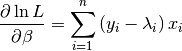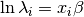# statsmodels.discrete.discrete_model.Poisson.score¶

Poisson.score(params)[source]

Poisson model score (gradient) vector of the log-likelihood

Parameters: params : array-like The parameters of the model score : ndarray, 1-D The score vector of the model, i.e. the first derivative of the loglikelihood function, evaluated at params

Noteswhere the loglinear model is assumed